Win up to 100% scholarship on Aakash BYJU'S JEE/NEET courses with ABNAT Win up to 100% scholarship on Aakash BYJU'S JEE/NEET courses with ABNAT

# Energy

## What Is Energy?

In recent days, we often hear about energy. Every invention and civilisation is based upon acquiring and using energy effectively. This is possible by the unique property of our Universe that it can transfer and transform
energy, but the total amount is always the same (conserved).

One fundamental focus of physics is to investigate energy. Energy in physics can be generally defined as a scalar quantity associated with the state or condition of one or more objects.

### Units of Energy

As per the International System of Units (SI), the unit of energy is said to be Joule (J). In addition to this, energy is also expressed in many other units which do not belong to the SI system. Some of these units include calories, ergs,  kilowatt-hours, kilocalories and British Thermal Units. However, these will need a conversion factor, if we want to express them in SI units. All of these are used in certain areas of commerce and science.

## Types of Energy

In this chapter, we will mainly focus on two forms of mechanical energy: kinetic and potential energy.

### Kinetic Energy

It is associated with the state of motion of an object. The greater the kinetic energy, the faster it moves. For an object of mass m and velocity v, it is given by the expression,

$$\begin{array}{l}K = \frac{1}{2}mv^{2}\end{array}$$

Example of Kinetic Energy

A 3 kg block moving past you at 2.0m/s has a kinetic energy of 6.0J.

### Potential Energy

It is associated with the configuration (arrangement) of the system of objects that exert forces on one another.

Example of Potential Energy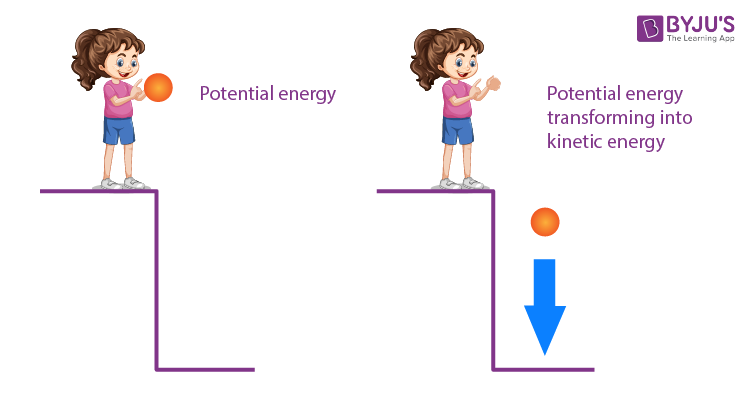When you throw a ball down from a height h above the surface of the earth, the increase in kinetic energy of the ball is accounted for by defining gravitational potential energy. This energy is associated with the state of separation (configuration) between two objects (ball and earth) attracted by gravitational force.

## Work and Potential energy

In the above example, the work done by the gravitational force is negative if the ball is thrown up. As the ball rises, its velocity (kinetic energy) decreases and reaches zero when it attains the maximum height. Now, we can tell that the energy the ball possesses at the maximum height is gravitational potential energy.

The work done by the gravitational force is stored as the potential energy. Hence, for any case, the change in potential energy is defined as being equal to the negative of the work done on the system.

$$\begin{array}{l}\Delta U = -W = -F.\Delta x\end{array}$$

Where F is the constant force, and Δx is the displacement due to the force.

More generally, if the force is a conservative force, then the change in potential energy is given by,

$$\begin{array}{l}\Delta U = \int_{r_1}^{r_2}F(r)dr\end{array}$$

Conservative force from potential energy can be found by,

$$\begin{array}{l}F(r) = -\frac{dU}{dx} = 0\end{array}$$

### Potential Energy and Equilibrium

The object is said to be in equilibrium if the net force acting on it is equal to zero.

$$\begin{array}{l}F(r) = -\frac{dU}{dx} = 0\end{array}$$

Stable equilibrium: A body is in stable equilibrium if it comes back to its normal position on slight displacement. For slight displacement, the restoring force should act on it.

$$\begin{array}{l}\frac{d^{2}U}{dx^{2}}> 0\end{array}$$

Unstable equilibrium: A body is in unstable equilibrium if it does come back to its normal position on slight displacement. For slight displacement, the restoring force should take away the object.

$$\begin{array}{l}\frac{d^{2}U}{dx^{2}}< 0\end{array}$$

Neutral equilibrium: We can move the particle slightly away from such a point, and it will still remain in equilibrium (i.e., it will neither attempt to return to its initial state nor will it continue to move).

$$\begin{array}{l}\frac{d^{2}U}{dx^{2}}= 0\end{array}$$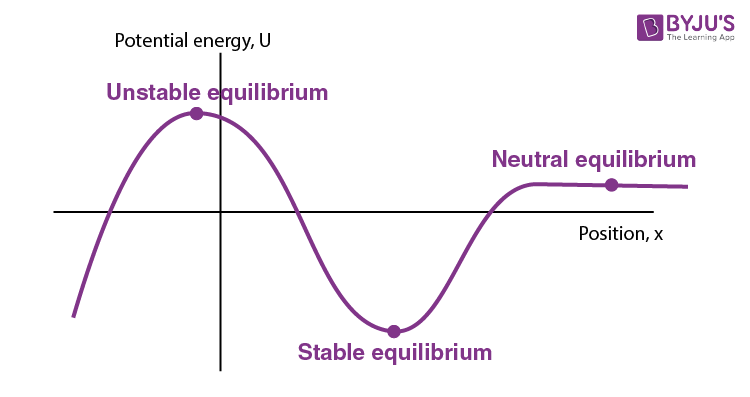Problem:

The potential energy of a conservative system is given by U = ax2– bx, where a and b are positive constants.

 This problem is an application of potential energy and conservative force relation.

Find the equilibrium, position and discuss whether the equilibrium is stable, unstable or neutral.In a conservation field

$$\begin{array}{l}F = \frac{dU}{dx}\end{array}$$
$$\begin{array}{l}\therefore F = -\frac{d}{dx}(ax^{2}-bx) = b-2ax\end{array}$$

For equilibrium F = 0 or b – 2ax = 0

$$\begin{array}{l}\therefore x = \frac{b}{2a}\end{array}$$

From the given equation, we can see that

$$\begin{array}{l}\frac{d^{2}U}{dx^{2}} = 2a\end{array}$$
(positive)., U is the minimum.

$$\begin{array}{l}\text{Therefore},\ x= \frac{b}{2a}\ \text{is the stable equilibrium position.}\end{array}$$

## Gravitational Potential Energy

The work done by the gravitational force for displacing the object from height 0 to h is given by,

$$\begin{array}{l}W = \int_{r_1}^{r_2}F.dr = \int_{0}^{h}mg \;dy\end{array}$$

$$\begin{array}{l}\Delta U = -\int_{0}^{h}-mg\;dy\end{array}$$

$$\begin{array}{l}\Delta U = mgh\end{array}$$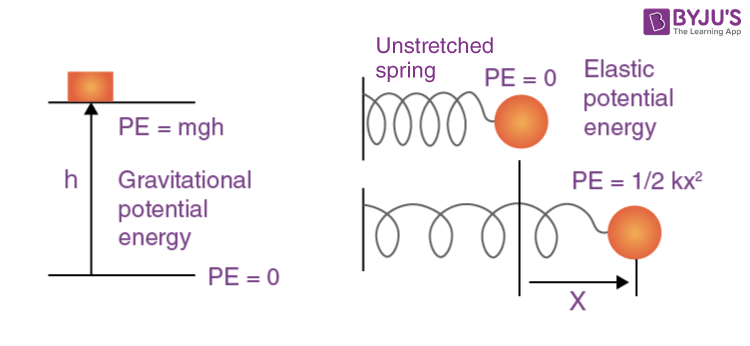## Elastic Potential Energy

Let’s consider a spring-mass system with a spring of spring constant k and a block of mass m displaced through a distance x from its equilibrium position (x=0). As the block moves from x=0 to x, the spring force, Fs=-kx does work on the block. The corresponding change in elastic potential energy is given by,

$$\begin{array}{l}\Delta U = -\int_{0}^{x}-kx\;dx\end{array}$$

$$\begin{array}{l}\Delta U = k[\frac{x^{2}}{2}]\end{array}$$

Problem:

A block of mass m is attached to two unstretched springs of constant k₁ and k₂, as shown in the figure. The block is displaced towards the right through a distance of x and released. Find the speed of the block as it passes through a distance x/4 from its mean position.

Applying conservation energy,$$\begin{array}{l}\frac{1}{2}K_1x^{2}+\frac{1}{2}K_2x^{2} = \frac{1}{2}mv^{2}\end{array}$$

$$\begin{array}{l}\frac{1}{2}k_1(x/4)^{2}+\frac{1}{2}k_2(x/4)^{2} = \frac{1}{2}mv^{2}\end{array}$$

$$\begin{array}{l}v= \frac{x}{4m}\sqrt{(k_1+k_2)}\end{array}$$

## Work-energy Theorem

This theorem states that work done by all the forces acting on a particle or body is equal to the change in its kinetic energy.

Let us take an example in which a block of mass m kept on a rough horizontal surface is acted upon by a constant force F parallel to the surface, and it is displaced through x. The initial velocity and the final velocity are, respectively, v0 and v.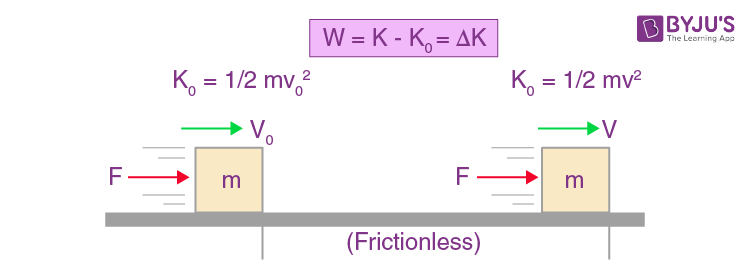Now, we apply Newton’s law.

$$\begin{array}{l}F = ma = m\left ( \frac{v^{2}-v_{o}^{2}}{2x} \right )\end{array}$$

Finally, the work done is

$$\begin{array}{l}W = Fx = m\left ( \frac{v^{2}-v_{o}^{2}}{2x} \right )x =\frac{1}{2}mv^{2}-\frac{1}{2}mv_{o}^{2}\end{array}$$

Now, we define the kinetic energy (K) of the object.

$$\begin{array}{l}K = \frac{1}{2}mv^{2}\end{array}$$

By this definition, the work done on the object is simply equal to the change in the object’s

$$\begin{array}{l}W = K-K_o = \Delta K\end{array}$$

Problem:

1. The displacement of a body in a metre is a function of time according to x = 2t4+5. The mass of the body is 2kg. What is the increase in its kinetic energy one second after the start of motion?

a) 8J

b) 16J

c) 32J

d) 64J

Solution : d

X = 2t4 +5

$$\begin{array}{l}\Rightarrow v = \frac{dx}{dt} = 8t^{3}\end{array}$$

$$\begin{array}{l}a = \frac{dv}{dt} = 24t^{2}\end{array}$$

$$\begin{array}{l}\Rightarrow F = ma = 48t^{2}\end{array}$$

$$\begin{array}{l}dW = Fdx = 48t^{2}\times8t^{3}dt\end{array}$$

An increase in the kinetic energy results from the work done by the applied force.

$$\begin{array}{l}\Delta KE = \int_{0}^{1} 48\times 8t^{5}dt = \frac{48\times 8}{6}t^{6} = \frac{48\times 8}{6} = 64\ J\end{array}$$

2. A 2kg block is placed on a frictionless horizontal surface. A force shown in the F – x graph is applied to the block horizontally. The change in kinetic energy is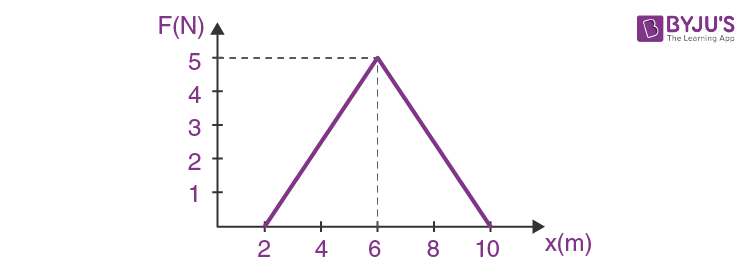a) 15J

b) 20J

c) 25J

d) 30J

Solution :

Work done = Area under F-x graph

W = (1/2) × (10 – 2) × 5 = 20J

Work done = Change in kinetic energy = 20J

### Law of Conservation of Energy

Conservation of energy means conservation of all forms of energy together. Accounting for all forms of energy within an isolated system, the total energy remains constant. Mechanical energy accounts for only two forms of energy, namely kinetic energy, K and potential energy, U.

If only conservative forces act on a system, then the total mechanical energy of the system remains constant. i.e.,

$$\begin{array}{l}K+U = constant\end{array}$$Example

Consider the case of a simple pendulum of a massive bob hanging at the end of a massless rod that is pivoted at a fixed point. If the pendulum is given a velocity from its equilibrium position, it executes a simple harmonic motion where energy is conserved at all points.

As the bob is raised from the equilibrium point, its position above the surface of the earth is increased, and hence, the potential energy is increased. It is accounted for the decrease in kinetic energy as the bob slows down while rising.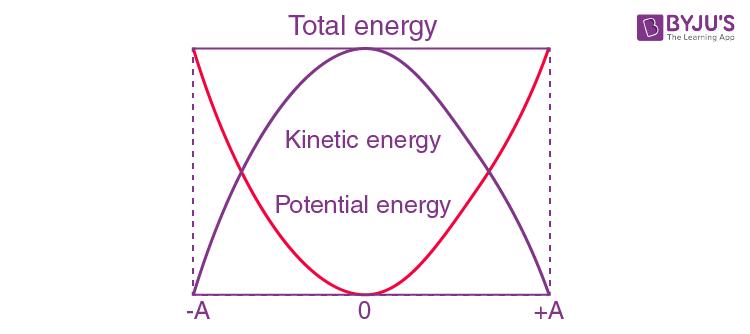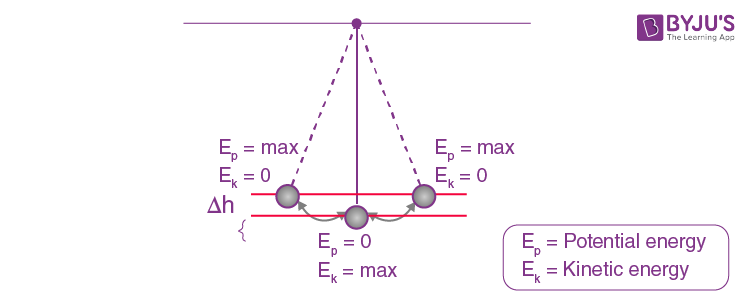When kinetic energy is maximum (at equilibrium position), potential energy is minimum. When potential energy is maximum (extreme positions), kinetic energy is minimum. Thus, at all points, total mechanical energy is conserved.

Problem:

1. Just before striking the ground, a 2.0kg mass has 400J of kinetic energy. If friction can be ignored, from what height was it dropped? (g = 9.8m/s2)

By conservation of mechanical energy,

Uf + Kf = Ui + Ki

or, 0 + Kf = mgh + 0

$$\begin{array}{l}\Rightarrow h=\frac{K_f}{mg} = \frac{400}{2\times9.8} = 20.4m\end{array}$$

2. An ideal massless spring ‘S’ can be compressed 1.0m by a 100N force. It is placed, as shown, at the bottom of a frictionless inclined plane, which makes an angle of θ = 300, horizontally. A 10 kg block is released from the top of the incline and is brought to rest momentarily after compressing the spring 2.0m. Through what distance does the mass slide before coming to rest?Spring constant, K= 100 N/m

The mass describes a distance x before reaching the spring. Then the total distance described is (x + 2) before coming to rest.

Work done by gravitational pull = 10 g(x + 2) sin 300

= 5 g(x + 2)

Work done by the elastic force of the spring = – (1/2) kx2

= – (1/2) x 100 x 22 = – 200

Net work done on the body = 5g (x + 2) – 200

Work energy theorem Δk = 0

Therefore, 5g (x + 2) – 200 = 0

x + 2 = [200/(5 x 10)]

x + 2 = 4

x = 2 along the inclined surface

## Frequently Asked Questions on Energy

Q1

### What is the unit of energy?

Joule is the unit of energy.

Q2

Potential energy
Kinetic energy

Q3

### What is the work-energy theorem?

According to the work-energy theorem, the net work done on an object by force equals its kinetic energy change.